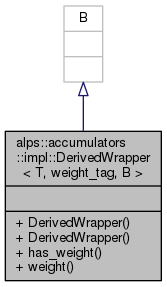ALPSCore reference
alps::accumulators::impl::DerivedWrapper< T, weight_tag, B > Class Template Reference

#include <weight.hpp>

Inheritance diagram for alps::accumulators::impl::DerivedWrapper< T, weight_tag, B >:Collaboration diagram for alps::accumulators::impl::DerivedWrapper< T, weight_tag, B >:## Public Member Functions

DerivedWrapper ()

DerivedWrapper (T const &arg)

bool has_weight () const

base_wrapper< typename value_type< T >::type > const * weight () const

## Detailed Description

### template<typename T, typename B> class alps::accumulators::impl::DerivedWrapper< T, weight_tag, B >

Definition at line 83 of file weight.hpp.

## Constructor & Destructor Documentation

template<typename T , typename B >
 alps::accumulators::impl::DerivedWrapper< T, weight_tag, B >::DerivedWrapper ( )
inline

Definition at line 85 of file weight.hpp.

template<typename T , typename B >
 alps::accumulators::impl::DerivedWrapper< T, weight_tag, B >::DerivedWrapper ( T const & arg )
inline

Definition at line 86 of file weight.hpp.

## Member Function Documentation

template<typename T , typename B >
 bool alps::accumulators::impl::DerivedWrapper< T, weight_tag, B >::has_weight ( ) const
inline

Definition at line 88 of file weight.hpp.

template<typename T , typename B >
 base_wrapper::type> const* alps::accumulators::impl::DerivedWrapper< T, weight_tag, B >::weight ( ) const
inline

Definition at line 89 of file weight.hpp.

The documentation for this class was generated from the following file: